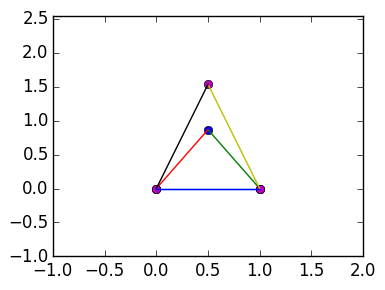# Lab 5 Stable Structures¶

We finish with the structure project in this lab. We will set up the linear system relating displacement of the nodes with forces on the nodes coming from the bars. We will then see this equation is underdetermined: the resulting matrix in the linear system has a non-trivial kernel. This is due to the structure "floating in space": we must fix nodes to stabilize the structure.

## Notation from previous labs¶

Recall that the nodes are a sequence of $n$ points in 2D:

$$\mathbf{a}_1=(x_1,y_1),\mathbf{a}_2=(x_2,y_2),\ldots,\mathbf{a}_n=(x_n,y_n).$$

The bars are then given by a sequence of $m$ pairs of nodes:

$$\mathbf{b}_1=(p_1,q_1),\mathbf{b}_2=(p_2,p_2),\ldots,\mathbf{a}_n=(p_n,q_n),$$

where $p_j$ and $q_j$ are integers between 1 and $n$. For example, the bar attaching $\mathbf{a_2}$ to $\mathbf{a_4}$ is represented as $(1,4)$.

A Structure S has two fields: nodes, which are a $2 \times m$ matrix

$$S.nodes=\begin{pmatrix} x_1 & x_2 & \cdots & x_n \cr y_1 & y_2 & \cdots & y_n \end{pmatrix}$$

and bars, a $2 \times m$ matrix

$$S.bars=\begin{pmatrix} p_1 & p_2 & \cdots & p_n \cr q_1 & q_2 & \cdots & q_n \end{pmatrix}$$

Therefore, elongations will be represented by a a vector of length $m$, one for each bar:

$$\mathbf{e} = \begin{pmatrix} e_1 \cr e_2 \cr \vdots \cr e_m\end{pmatrix}$$

The bars obey hooks law, where we take the stiffness of each bar to be one. Therefore the magnitude of the force for each bar is $y_k = e_k$, or in vector for m $\mathbf y = \mathbf e$.

We will represent the normal directions coresponding to the bars of a Structure by a $2 \times m$ matrix:

$$N:=\left(\mathbf{n}(\mathbf{a}_{p_1},\mathbf{a}_{q_1}) \ |\ \mathbf{n}(\mathbf{a}_{p_2},\mathbf{a}_{q_2})\ |\ \cdots\ |\ \mathbf{n}(\mathbf{a}_{p_m},\mathbf{a}_{q_m}) \right) = \left(\mathbf{n}_1\ |\ \mathbf{n}_2\ |\ \cdots\ |\ \mathbf{n}_m \right) = \begin{pmatrix} n_1^x & n_2^x & \cdots & n_m^x \cr n_1^y & n_2^y & \cdots & n_m^y \end{pmatrix}$$

where $$\mathbf{n}(\mathbf{c},\mathbf{d}) := {\mathbf{c} - \mathbf{d} \over \|\mathbf c - \mathbf d\|}$$

The external forces will be given by vectors $\mathbf{f}_1$, $\mathbf{f}_2$, …, $\mathbf{f}_n$, one external force per node. They will be represented by the $2 \times n$ matrix

$$F := \left(\mathbf f_1\ |\ \cdots\ |\ \mathbf f_n \right) = \begin{pmatrix} f_1^x & f_2^x & \cdots & f_n^x \cr f_1^y & f_2^y & \cdots & f_n^y \end{pmatrix}$$

For setting up linear systems, this can be vectorized as

$$\mathbf f = vec(F) = \begin{pmatrix} f_1^x \cr f_1^y \cr f_2^x \cr f_2^y \cr \vdots \cr f_n^x \cr f_n^y\end{pmatrix}$$

We want to model the displacement $\mathbf{u}_k$ of each node $\mathbf{a}_k$ under forces, or as a matrix

$$U =\left(\mathbf u_1\ | \ \cdots \ |\ \mathbf u_n \right)= \begin{pmatrix} u_1^x & \cdots & u_n^x \cr u_1^y & \cdots &u_n^y \end{pmatrix}$$

This can also be vectorized as

$$\mathbf u = vec(U) = \begin{pmatrix} u_1^x \cr u_1^y \cr u_2^x \cr u_2^y \cr \vdots \cr u_n^x \cr u_n^y\end{pmatrix}$$

# Setup code¶

First, evaluate the necessary functions from the previous labs:

In :
using PyPlot
import PyPlot.plot

type Structure
nodes::Matrix{Float64}
bars::Matrix{Int64}
end

function Structure(nodes::Matrix{Float64})
bars=zeros(Int,2,0)  # creates a 0 x 2 array
Structure(nodes,bars)
end

numnodes(S::Structure)=size(S.nodes,2)
numbars(S::Structure)=size(S.bars,2)

oldbars=S.bars
m=numbars(S)
newbars=zeros(2,m+1)

newbars[:,1:m]=oldbars
newbars[:,m+1]=[k,j]

S.bars=newbars  # replace S.bars with the newbars
S   # return S
end

function nodes(S::Structure,k)
S.nodes[:,S.bars[1,k]],S.nodes[:,S.bars[2,k]]
end

function plot(S::Structure)
A=S.nodes

# plot the nodes
x=vec(A[1,:])
y=vec(A[2,:])

plot(x,y;marker="o",linestyle="")

## TODO: plot bars
for k=1:numbars(S)
a,b=nodes(S,k)
plot([a,b],[a,b])
end

# the following code changes the axis to
minx=minimum(A[1,:])
maxx=maximum(A[1,:])

miny=minimum(A[2,:])
maxy=maximum(A[2,:])

axis([minx-1,maxx+1,miny-1,maxy+1])
end

normal(a,b)=(a-b)/norm(a-b)
function normals(S::Structure)
N=zeros(Float64,2,numbars(S))

for k=1:numbars(S)
a,b=nodes(S,k)
N[:,k]=normal(a,b)
end
N
end

function plotnormals(S::Structure)
N=normals(S)

for k=1:numbars(S)
a,b=nodes(S,k)

end
end

function plotbarforces(S::Structure,e::Vector{Float64})
N=normals(S)

for k=1:numbars(S)
a,b=nodes(S,k)

end
end

function plotexternalforces(S::Structure,F::Matrix)
for k=1:size(F,2)
a=S.nodes[:,k]

end
end

function incidentmatrices(S::Structure)
m=numbars(S)
n=numnodes(S)
B=S.bars

Mx=zeros(m,n)
My=zeros(m,n)
N=normals(S)
for k=1:m   # populate e_k
nx,ny=N[:,k]
Mx[k,B[1,k]]=nx
My[k,B[1,k]]=ny
Mx[k,B[2,k]]=-nx
My[k,B[2,k]]=-ny
end

Mx,My
end

function incidentmatrix(S::Structure)
Mx,My=incidentmatrices(S)

M=zeros(size(Mx,1),2size(Mx,2))
for k=1:size(Mx,2)
M[:,2k-1]=Mx[:,k]
M[:,2k]=My[:,k]
end

M
end

Out:
incidentmatrix (generic function with 1 method)

# Displacements¶

We will now setup displacement of a structure. Recall that the displacements are given by a $2 \times n$ matrix $U$, where the $k$th column gives the displacement of the $k$th node.

Exercise 1(a) Create a one-line function called displace(S::Structure, U::Matrix) that returns a new Structure, whose nodes are equal to S.nodes+U and bars equal to S.bars

In :
displace(S::Structure,U::Matrix)=Structure(S.nodes+U,S.bars)

Out:
displace (generic function with 1 method)

1(b) Ensure the following plots correctly:

In :
S=Structure([1/2. 1. 0.; sqrt(3)/2. 0. 0.], [1 3 2; 2 1 3])

U=[-0.1 0.2 -0.1; 0.2 -0.1 -0.2]

S_displaced=displace(S,U)

plot(S)
plot(S_displaced);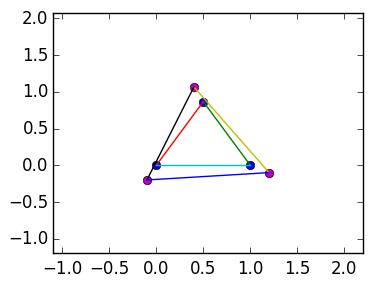1(c) Look-up ?reshape.

In :
?reshape

search:
Out:
reshape(A, dims)

Create an array with the same data as the given array, but with different dimensions. An implementation for a particular type of array may choose whether the data is copied or shared.

reshape promote_shape



1(d) For u=[1,2,3,4,5,6], figure out how to use reshape to create a matrix U = [1 3 5; 2 4 6].

In :
u=[1,2,3,4,5,6]
reshape(u,2,3)

Out:
2x3 Array{Int64,2}:
1  3  5
2  4  6

1(e) Use reshape and the previous displace command to write a function displace(S::Structure,u::Vector) so that displace(S,vec(U)) returns the same structure as displace(S,U). (Hint: you'll need to use numnodes.)

In :
displace(S::Structure,u::Vector)=displace(S,reshape(u,2,numnodes(S)))

Out:
displace (generic function with 2 methods)

1(f) Ensure that the following plots the same as before:

In :
S=Structure([1/2. 1. 0.; sqrt(3)/2. 0. 0.], [1 3 2; 2 1 3])
U=[-0.1 0.2 -0.1; 0.2 -0.1 -0.2]

u=vec(U)

S_displaced=displace(S,u)

plot(S)
plot(S_displaced)Out:
4-element Array{Float64,1}:
-1.1
2.2
-1.2
2.06603

# Incident matrix¶

We recall the definition of an incident matrix, relating the elongations to the displacements:

$$\mathbf e = M \mathbf u$$

for the incident matrix $M$, given by the command incidentmatrix.

Excercise 2(a) Use plotbarforces(S,e) and incendentmatrix(S) to plot the forces on the nodes induced by the displacement U as specified below:

In :
S=Structure([1/2. 1. 0.; sqrt(3)/2. 0. 0.], [1 3 2; 2 1 3])
U=[-0.1 0.2 -0.1; 0.2 -0.1 -0.2]
M=incidentmatrix(S)

e=M*vec(U)

plot(S)
plot(displace(S,U))
plotbarforces(S,e)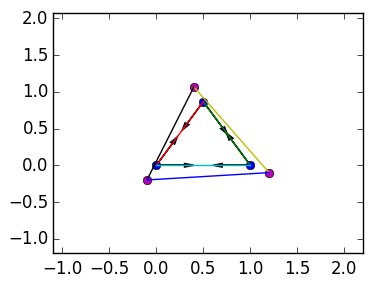In this simple example, we take every bar to have the same stiffness of 1, so that the magnitude of the forces equals the displacement: $\mathbf y = \mathbf e$. We now want to determine the total forces from the bars induced from an elongation: that is, a sum over the force vectors from plotbarforces. This is given by

$$\sum_{j=1}^m \begin{cases} y_j \mathbf{n}_j & if p_j = k \cr -y_j \mathbf{n}_j & if q_j = k \cr 0 & otherwise \end{cases}$$

2(b) (Optional) Show that if the bars are elongated by $\mathbf e$, then the total force exhibited by the bars is given by

$$-M^\top \mathbf e$$

2(c) Plot the total forces for the following S and U. (Hint: they should point into the triangle.)

In :
S=Structure([1/2. 1. 0.; sqrt(3)/2. 0. 0.], [1 3 2; 2 1 3])
U=[-0.1 0.2 -0.1; 0.2 -0.1 -0.2]

e=M*vec(U)

f=-M'*e
F=reshape(f,2,3)

plot(S)
plotbarforces(S,e)
plotexternalforces(S,F)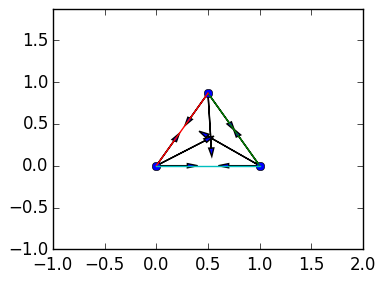# Forces sum to zero¶

The forces on each node must sum to zero for the structure to be in equilibrium. This means that we require the forces from the bars to equal the external forces given by a matrix $F$. In otherwords, we have the condition

$$0 = \mathbf f - M^\top \mathbf e$$

Or, the linear system

$$\mathbf f = M^\top \mathbf e = M^\top M \mathbf u = K \mathbf u$$

There is a catch: the matrix $K$ is not invertible. This is an artifact of the structure floating in space: there are many possible stable solutions, corresponding to translations and rotations.

Exercise 3(a) Use rank to determine the rank of K=M'*M.

In :
K=M'*M
rank(K)

Out:
3

3(b) What displacements $\mathbf v$ and $\mathbf h$ correspond to vertical and horizontal translations?

3(c) Verify numerically that $M\mathbf v$ and $M \mathbf h$ are zero. Why do vertical and horizontal translations result in no elongation? What does this say about $K\mathbf v$ and $K \mathbf h$? Test this hypothesis numerically.

In :
v=[1,0,1,0,1,0]
h=[0,1,0,1,0,1]
M*h

Out:
3-element Array{Float64,1}:
0.0
0.0
0.0

A third element of the kernel corresponds to rotating all nodes at the same time. A rotation that fixes the bottom left node gives rise to the kernel element

$$\mathbf r = \begin{pmatrix} -\sqrt 3/2\cr 1/2\cr 0\cr 1\cr 0\cr0 \end{pmatrix}$$

3(d) Verify numerically that $M\mathbf r$ is equal to zero.

In :
r=[-sqrt(3)/2,1/2,0,1,0,0]
M*r

Out:
3-element Array{Float64,1}:
1.11022e-16
-5.55112e-17
0.0        

3(e) Plot the structure that results by the displacement $0.1\mathbf r$, $0.2\mathbf r$ and $0.3\mathbf r$. Does this displacement continue to correspond to rotation as the magnitude becomes large?

In :
plot(S)
plot(displace(S,0.1r))
plot(displace(S,0.2r))
plot(displace(S,0.3r))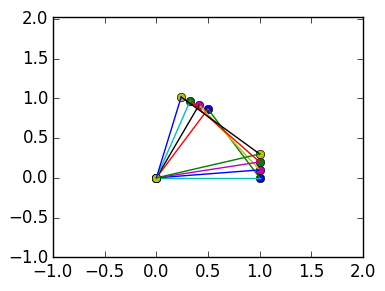Out:
4-element Array{Float64,1}:
-1.0
2.0
-1.0
2.01603

## The nullspace¶

Above, we have constructed the 3-dimensional kernel of $M$:

$$Z:= \begin{pmatrix} 1 & 0 & -\sqrt 3/2\cr 0 & 1 & 1/2\cr 1 & 0 & 0\cr 0 & 1 & 1\cr 1 & 0 & 0\cr0 & 1 & 0 \end{pmatrix}$$

We will compare this exact kernel to the one generated by the inbuilt nullspace command.

Exercise 4(a) Check numerically that M*Z = zeros(3,3)

In :
Z=[1 0 -sqrt(3)/2;  0 1 1/2; 1 0 0; 0 1 1; 1 0 0; 0 1 0]

M*Z

Out:
3x3 Array{Float64,2}:
0.0  0.0   1.11022e-16
0.0  0.0  -5.55112e-17
0.0  0.0   0.0        

4(b) Use nullspace to construct another $6 \times 3$ matrix Q so that M*Q = \zeros(3,3). Is Q approximately Z?

In :
Q=nullspace(M)

Out:
6x3 Array{Float64,2}:
-0.605994    0.377905    0.39576
0.343331    0.0700097   0.458863
0.0377708   0.632207   -0.124718
0.715009    0.216831    0.158365
0.0377708   0.632207   -0.124718
-0.0283465  -0.0768116   0.759361

4(c) Show numerically that Q is orthogonal. (Recall the definition of orthogonal for rectangular matrices)

In :
Q'*Q

Out:
3x3 Array{Float64,2}:
1.0          -3.64292e-17  -1.21431e-16
-3.64292e-17   1.0          -6.93889e-18
-1.21431e-16  -6.93889e-18   1.0        

4(d) Since Q is rectangular, x=Q\b gives the least squares approximation. For b=rand(6), Show numerically that x is approximately equal to Q'*b. Why is this true?

In :
b=rand(6)
x=Q\b
norm(Q'*b-x)

Out:
2.482534153247273e-16

4(e) Show numerically that the columns of Q and Z span the same space. (Hint: If b lies in the span of the columns of Q, then norm(Q*x-b) must be zero.)

In :
norm(Q*Q'*Z-Z)

Out:
9.504445130003892e-16

## QR Decomposition and nullspace¶

Exercise 5 (a) (Advanced) Inspect Q,R=qr(K). What do you notice about the zero entries of R?

5(b) Use the observation about the zeros of R to construct the kernel of K from Q. (Hint: K is symmetric.)

In :
K=M'*M

Q,R=qr(K)

Out:
(
6x6 Array{Float64,2}:
-0.57735    9.71154e-18   0.0        0.407876   -0.207066    0.676334
0.0       -0.774597      0.258199   0.0246581  -0.547223   -0.182408
0.288675  -0.223607     -0.67082    0.631815    0.0665457  -0.114228
-0.5        0.387298     -0.129099   0.15395    -0.389253   -0.638839
0.288675   0.223607      0.67082    0.631815    0.0665457  -0.114228
0.5        0.387298     -0.129099  -0.104633   -0.705192    0.274023,

6x6 Array{Float64,2}:
-0.866025   0.0       0.433013  -0.75          0.433013      0.75
0.0       -1.93649  -1.00623    0.968246      1.00623       0.968246
0.0        0.0      -1.34164    1.11022e-16   1.34164       1.11022e-16
0.0        0.0       0.0       -4.58437e-17  -2.22045e-16  -6.87655e-17
0.0        0.0       0.0        0.0           6.20634e-17  -5.91931e-17
0.0        0.0       0.0        0.0           0.0          -1.02482e-16)

## Stabilizing the structure¶

The previous structure was not stable because it was floating in space. We can make it stable by fixing the bottom two nodes on the ground. This results in no forces on the bottom two nodes, but now the displacements are enforced to be zero.

Exercise 6 (Advanced) (a) The 3rd through last rows of $K \mathbf u = \mathbf f$ correspond to the forces on the bottom two nodes. Modify these rows of the equation to instead specify that these two nodes are not displaced:

$$\mathbf u[3:end] = 0$$

(Hint: you want to add identity matrices.)

(b) Use rank to show that the equation is now solveable.

(c) Ensure that the following code plots the solution correctly:

In :
M=incidentmatrix(S)
K=M'*M

K[3:end,:]=zeros(4,6)
K[3:4,3:4]=eye(2)
K[5:6,5:6]=eye(2)

u=K\[0.,1.,0.,0.,0.,0.]

plot(S)
plot(displace(S,u));